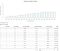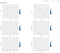# Build a Shiny WebApp from ScratchPhoto by Carlos Muza on Unsplash

As a school project for the course of MonteCarlo Simulation, I built a Shiny WebApp with my team from scratch: Clinic Sunday Operation Simulation WebApp. If you know basics of coding, but got intimidated by the idea of building an WebApp all by yourself like me(I know absolute nothing about WebApp 5 weeks ago), then this article is for you.

Shiny Package (R) is a very cool tool, which takes a interactive approach to telling the data story. I familiarized myself with Shiny Basics by taking a quick course from DataCamp: Building Web Applications with Shiny in R. And then I started my journey with trials and errors.

Before diving deep into the technical part, here just brief summarizes the background: the project hypothetically constructed a eye clinic which has been considering Sunday operations. The Shiny App is built to facilitate the manager’s decision making, which allows managers to try out different combinations of schedule intervals (mins) and doctor’s hourly salary. The tool will simulate the clinic’s typical day operation and provide the key performance metrics: profit, average waiting time (scheduled and walk-ins) and doctor’s overtime based on different decisions as outputs.

Here are screenshots of the finished WebApp:Simulation TabAll Combination tab

To build a such WebApp that allows user to interact with data does not even require any deep knowledge of html or CSS. Here are the steps I followed:

Before hit the ground running, think about the goal and key components of the tool:

• What are the inputs and expected outputs? Which inputs are expected from users and which are fixed?
• What are the key functions you want to realize? (the engine link inputs and outputs)
• How do you want to organize the components ?(how many tabs do you want to split?)

I started my first line of code just on a regular .r file, because I just wanted to try out the functions in a familiar way. In this step, you can just forget Shiny for a While, just focusing on writing up the functions.

Since I have two long explanatory paragraphs (introduction & analysis), I created a simple .html file for each as below, so that I don’t need to keep long words or format in UI.

`<br/><h3>Introduction</h3><p>We hypothetically constructed a clinic that provides two types of services: eye examination (does not need reservation) and cataract surgery services (needs reservation). Now the clinic operates from Monday to Saturday. To cater to greater market demand, the clinic's manager has been considering Sunday operations.</p><p>However, before hitting the ground and running, the manager needs to make two decisions: </p>...`

With the .r engine and .html write-up, I have the all the flesh (content), then I will need skeletons (UI/Server) to support the system.

OK, now we need to wrap the ready-built engine with Shiny.

First we need to install and import the Shiny package, then you can create a shiny app from them R-Studio template:

`library(shiny)`

Then we can see that the template already help us set up the main structure for our app:

`ui <- fluidPage(# Application title    titlePanel("Old Faithful Geyser Data"),# Sidebar with a slider input for number of bins     sidebarLayout(        sidebarPanel(            sliderInput("bins",                        "Number of bins:",                        min = 1,                        max = 50,                        value = 30)        ),# Show a plot of the generated distribution        mainPanel(           plotOutput("distPlot")        )    ))# Define server logic required to draw a histogramserver <- function(input, output) {output\$distPlot <- renderPlot({        # generate bins based on input\$bins from ui.R        x    <- faithful[, 2]        bins <- seq(min(x), max(x), length.out = input\$bins + 1)# draw the histogram with the specified number of bins        hist(x, breaks = bins, col = 'darkgray', border = 'white')    })}# Run the application shinyApp(ui = ui, server = server)`

After this, I copy paste my codes (main functions and fixed inputs) before the UI blocks.

Here are some building blocks of my UIs:

• I split my app into 5 tabs:
`ui <- fluidPage(# Application title    titlePanel("Clinic Sunday Operation Simulation"),          tabsetPanel(            tabPanel("Introduction & Model"),               tabPanel("Simulation"),            tabPanel("All Combinations"),            tabPanel("Analysis"),            tabPanel("About the Project")))`
• I insert the .html files and add simple words to the tabs (since this step is very straightforward)
`ui <- fluidPage(# Application title    titlePanel("Clinic Sunday Operation Simulation"),          tabsetPanel(            tabPanel("Introduction & Model",                     includeHTML("model_intro.html")),            tabPanel("Simulation"),            tabPanel("All Combinations"),            tabPanel("Analysis",                      includeHTML("analysis.html")),            tabPanel("About the Project",                     h4("Contact"),                     p("Julia Yuxiao Yang"),                     p("julia.yuxiao.yang@outlook.com"),                     hr(),                     h4("File Components:"),                     p("Clinic Project Shiny Version 1.R"),                     p("model_intro.html"),                     p("analysis.html"),                     hr())))`
• For the tabs that contains interactive elements (user input -> output), I want users to select the salary / intervals number by using sliders, so I define the input as:
`tabPanel("Simulation",          sliderInput("Schedual_Inverval","Schedual Inverval (mins):",min = 20,max = 60,value = 30,step = 5),          sliderInput("Salary","Doctor's Hourly Salary (\$):",min = 60,max = 140,value = 80,step = 20)# in this way, we tells the app that "Schedual_Inverval" & "Salary" are two inputs by users`
• I also used the structure as below (example) to leave room for the output text/plot/table:
`fluidRow(column(3, h4("Avg Profit")),         column(3, h4("Avg WaitTime-Scheduled")),         column(3, h4("Avg WaitTime-Walkin")),         column(3, h4("Avg Doctor Overtime"))),# xxx are to be updated once outputs in server are definedfluidRow(column(3, wellPanel (div(textOutput("xxx"),style = "font-size:100%"))),         column(3, wellPanel (div(textOutput("xxx"),style = "font-size:100%"))),         column(3, wellPanel (div(textOutput("xxx"),style = "font-size:100%"))),...`

To use the input variables that entered by users (selected by the sliders in my case) to generate outputs, we need to wrap them with Reactive ({}), for example:

`mdf1= reactive({df_func1(repsim(input\$Salary,input\$Schedual_Inverval))})# repsim & df_func1 are pre-defined functions# mdf1 is a Dataframe`

To use the object that is determined by the input, we need to use the form of object():

` x <- mdf1()`

Here are some common outputs:

libraries:

`library(ggplot2)  #plotlibrary(DT) #tablelibrary(dplyr)library(plotly) #plot`
• Plots:
`# ggplot:output\$distPlot1 <- renderPlot({        x    <- mdf1()       ggplot(x, aes(x = x[,6]))  + geom_histogram(bins = 20,          color="darkblue", fill="lightblue") + labs(x = "Clinic Profit (\$) ", y = "Count", title = 'Clinic Profit Simulation Histogram')            })# plotly:  output\$plot_7<- plotly::renderPlotly({        # Plot for all combination simulation - Analysis Tab        plot_ly(            trail_df, x = ~Avg_Wait_Schedual, y = ~Profit,            # Hover text:            type   = 'scatter',             size = ~Over_time_Mins,            mode   = 'markers',            fill = ~'',            marker = list(sizemode = 'diameter')        )    })`
• Texts:
`# text: output\$profit <- renderText({        #Average profit        paste(round(mean(mdf1()\$Profit),2),'+/-',round(confidence_interval(mdf1()\$Profit,0.95),2))})`
• Tables:
`# table: (DT table)output\$table1<-  renderDT({            DT::datatable(mdf1(),editable = TRUE, options = list(paging = TRUE, searching = FALSE, ordering = FALSE, info = FALSE)) %>% formatRound(columns=c(3:6), digits=2) %>% formatStyle(columns=c(1:6), textAlign = 'center')        })`

In this step, we will need to update outputs to UI.

`fluidRow(column(3, wellPanel (div(textOutput("profit"),style = "font-size:100%"))),...`

Finally, once all outputs are updated to UI, the WebApp is Done! What we need to do is just run a few rounds and look for things for adjustments.

The next step would be to deploy the WebApp, I will write another story for that once my deployment is done :)

Master of Business Analytics of UBC. www.linkedin.com/in/julia-yuxiao-yang/